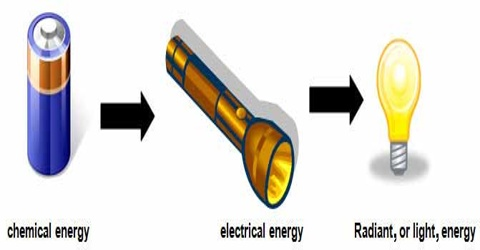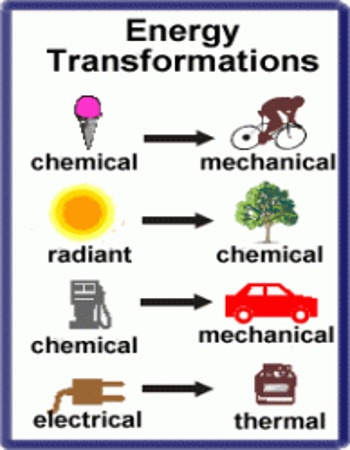# Transformation of Energy

Transformation of Energy

Energy is present in different forms in this universe. Different forms of energy are related to one other. It is possible to transform one form of energy to another form and it is called transformation of energy. Some examples of transformation of energy are given below:

(1) Water flows from higher position to a lower position. Its energy at higher place is potential energy. While flowing downward its potential energy changes to kinetic energy, by rotating turbines by this kinetic energy electricity is generated. That means mechanical energy has been transformed into electric energy.

(2) When electric energy passes through electric bulb, we get light. Here electric energy has been transformed into light energy.

(3) When current is passed through an electric iron, heat is produced. We iron clothes by this heat. Here electric energy has been converted to thermal energy.

(4) If an insulated cast iron is wrapped by copper wire and current is allowed to flow through it, then the iron plate becomes a magnet. Here electric energy is converted into magnetic energy.

(5) When light is incident on metals like calcium, potassium, rubidium etc., than electrons are emitted. Photo-electric cell is based on this principle. When light is incident on this type of cell current is produced. Here, light energy is convened into elector energy.(6) When two palms are rubbed with each other then heat is generated. In this case mechanical energy is converted into heat energy.

(7) When photographic film is exposed to light, then by chemical reaction photos are produced. Here light energy is transformed into chemical energy.

(8) In pharmaceutical industry germs and bacteria are destroyed by ultra- and infra-sound waves and naphtha is dissolved in water. Besides, clothes are cleaned by ultra-sound. Here sound energy is converted into mechanical energy.

(9) We know electric bells work by electricity. Telephone also works by electricity. In both cases we hear sound. Here electric energy is transformed into sound energy.

(10) When coal is burnt heat is produced. This happens due to chemical action. In this case chemical energy is transformed into heat energy.

(11) In electric cell electricity is produced due to chemical reaction. Here chemical energy is transformed into electric energy.

When energy is transformed from one form to another form, no loss or gain of energy occurs. That means, it is impossible to create or destroy energy. When one form of energy disappears it reappears in another form. This is called conservation of energy. There is a principle about it It is called conservation principle of energy. It is also called conservation law of energy.